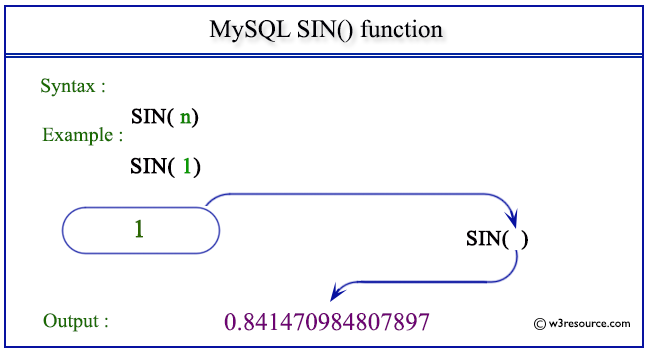# MySQL SIN() function

## SIN() function

MySQL SIN() returns the sine of the argument. The argument is given in radians.

This function is useful in -

• SIN() is a fundamental trigonometric function that is used extensively in mathematics and engineering to solve problems related to angles, distances, and periodic phenomena.
• It's essential in signal processing and waveform analysis.
• In geometry and physics, SIN() is crucial for calculating distances, angles, and trajectories.
• Engineers use SIN() for tasks like structural analysis, where understanding the behavior of materials under different loads and angles is essential.
• In computer graphics and animation, SIN() is used to generate smooth and realistic motion, especially in scenarios involving rotation and oscillation.
• It's a fundamental component of trigonometric identities and formulas, which are used to solve a wide range of mathematical problems.
• SIN() is used in various simulations to model natural phenomena, such as the motion of pendulums, the behavior of springs, and the propagation of waves.

Syntax:

```SIN(X);
```

Argument:

Name Description
X A number whose SIN value is to be retrieved.

Syntax Diagram:MySQL Version: 8.0

Pictorial presentation of MySQL SIN() functionExample of MySQL SIN() function

Code:

``````SELECT SIN(1);
```
```

Explanation:

The above MySQL statement will return the sine of 1.

Output:

```mysql> SELECT SIN(1);
+-------------------+
| SIN(1)            |
+-------------------+
| 0.841470984807897 |
+-------------------+
1 row in set (0.01 sec)
```

All Mathematical Functions

Previous: SIGN()
Next: SQRT()

﻿